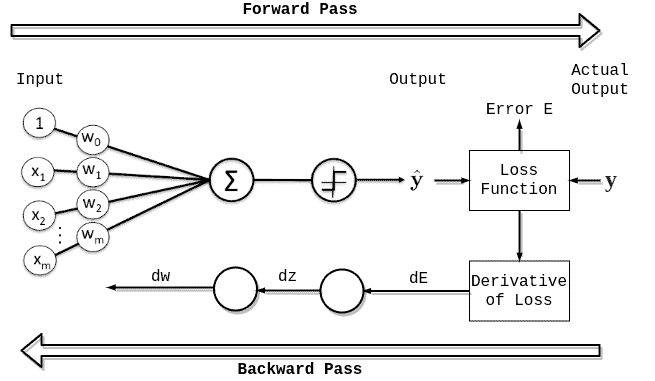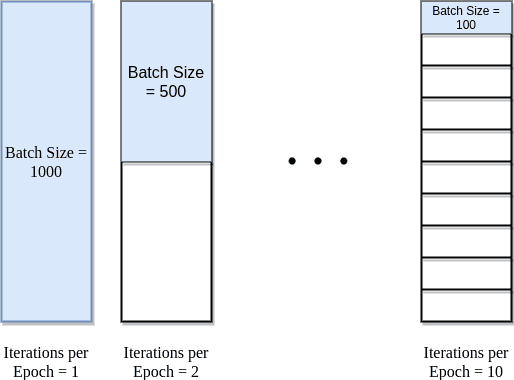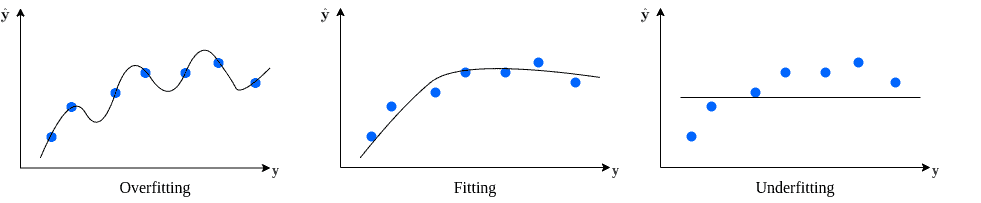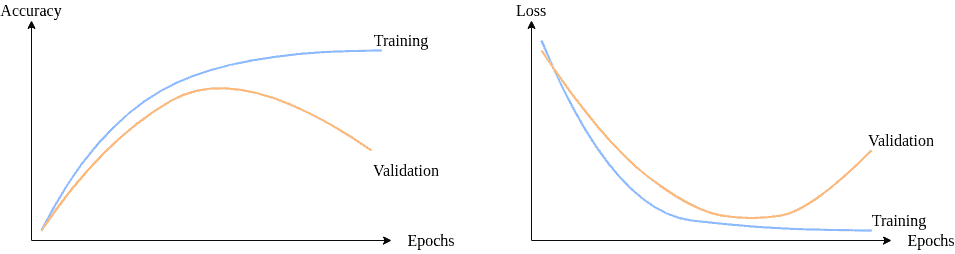## 1. Introduction

In this tutorial, we’ll learn about the meaning of an epoch in neural networks. Then we’ll investigate the relationship between neural network training convergence and the number of epochs. Finally, we’ll try to understand how we use early stopping to get better generalizing models.

## 2. Neural Networks

A neural network is a supervised machine learning algorithm. We can train neural networks to solve classification or regression problems. Yet, utilizing neural networks for a machine learning problem has its pros and cons.

Building a neural network model requires answering lots of architecture-oriented questions. Depending on the complexity of the problem and available data, we can train neural networks with different sizes and depths. Furthermore, we need to preprocess our input features, initialize the weights, add bias if needed, and choose appropriate activation functions.

## 3. Epoch in Neural Networks

An epoch means training the neural network with all the training data for one cycle. In an epoch, we use all of the data exactly once. A forward pass and a backward pass together are counted as one pass:An epoch is made up of one or more batches, where we use a part of the dataset to train the neural network. We call passing through the training examples in a batch an iteration.

An epoch is sometimes mixed with an iteration. To clarify the concepts, let’s consider a simple example where we have 1000 data points as presented in the figure below:If the batch size is 1000, we can complete an epoch with a single iteration. Similarly, if the batch size is 500, an epoch takes two iterations. So, if the batch size is 100, an epoch takes 10 iterations to complete. Simply, for each epoch, the required number of iterations times the batch size gives the number of data points.

We can use multiple epochs in training. In this case, the neural network is fed the same data more than once.

## 4. Neural Network Training Convergence

Deciding on the architecture of a neural network is a big step in model building. Still, we need to train the model and tune more hyperparameters on the way.

During the training phase, we aim to minimize the error rate as well as to make sure that the model generalizes well on new data. The bias-variance tradeoff is still a potential pitfall we want to avoid, as in other supervised machine learning algorithms.

We face overfitting (high variance) when the model fits perfectly to the training examples but has limited capability generalization. On the other hand, if the model is said to be underfitting (high bias) if it didn’t learn the data well enough:A good model is expected to capture the underlying structure of the data. In other words, it does not overfit or underfit.

When building a neural network model, we set the number of epochs parameter before the training starts. However, initially, we can’t know how many epochs is good for the model. Depending on the neural network architecture and data set, we need to decide when the neural network weights are converged.

For neural network models, it is common to examine learning curve graphs to decide on model convergence. Generally, we plot loss (or error) vs. epoch or accuracy vs. epoch graphs. During the training, we expect the loss to decrease and accuracy to increase as the number of epochs increases. However, we expect both loss and accuracy to stabilize after some point.

As usual, it is recommended to divide the data set into training and validation sets. By doing so, we can plot learning curve graphs for different sets. These graphs help us diagnose if the model has over-learned, under-learned, or fits the learning set.

We expect a neural network to converge after training for a number of epochs. Depending on the architecture and data available, we can treat the number of epochs to train as a hyperparameter.

Neural network weights are updated iteratively, as it is a gradient descent based algorithm. A single epoch in training is not enough and leads to underfitting. Given the complexity of real-world problems, it may take hundreds of epochs to train a neural network.

As a result, we expect to see the learning curve graphs getting better and better until convergence. Then if we keep training the model, it will overfit, and validation errors begin to increase:Training a neural network takes a considerable amount of time, even with the current technology. In the model-building phase, if we set the number of epochs too low, then the training will stop even before the model converges. Conversely, if we set the number of epochs too high, we’ll face overfitting. On top of that, we’ll waste computing power and time.

A widely adopted solution to this problem is to use early stopping. It is a form of regularization.

As the name suggests, the main idea in early stopping is to stop training when certain criteria are met. Usually, we stop training a model when generalization error starts to increase (model loss starts to increase, or accuracy starts to decrease). To decide on the change in generalization errors, we evaluate the model on the validation set after each epoch.

By utilizing early stopping, we can initially set the number of epochs to a high number. This way, we ensure that the resulting model has learned from the data. Once the training is complete, we can always check the learning curve graphs to make sure the model fits well.

## 5. Conclusion

In this article, we’ve learned about the epoch concept in neural networks. Then we’ve talked about neural network model training and how we can train models without overfitting or underfitting.